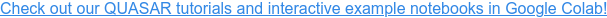QUASAR Examples

# QUASAR: Stochastic Programming in Python

In this blog post, I am going to show you just how easy it is to set up and solve a stochastic programming problem. For the example, I am going to use our Python interface that integrates seamlessly with widely used Python packages such as Numpy and Pandas. Let us begin by importing the needed packages into our work space.

In :
``` import pandas, quasar
```

### Data and Parameters¶

The decision problem that guides this example is a simple storage optimization model. The decision-maker must decide when and how much to inject and widthdraw from a storage. What makes the decision problem stochastic is that prices follow some random process and any decision taken now effects future opportunities to inject and withdraw as well as how profitable the storage will be. We are planning our storage over next 12 time periods (think of months) and need to account for capacity limits as well as possibly losses.

In :
``` time_horizon = 12
loss = 0.1
max_fill = 100
max_rate = 25
```

### Model Formulation¶

What is special about QUASAR is that you can formulate any stochastic programming problem just like it was a linear program, but unlike with conventional solvers, any model parameter can be a random variable. All it takes is using the keyword `rand` along with a string identifier that must be unique for a given stage.

In :
``` # decision model
model = quasar.DecisionProblem()
# starting state
s = {-1 : 25}
# multiple stages
for t in range(time_horizon):
# decision variables
# constraints
s[t] == s[t-1] + (1-loss)*c - r
# objective with random variable
model.obj += quasar.rand("price") * (r - c)
```

### Time Series Data¶

In the above example, prices are labeled as random variables. Now we need to define a stochastic process that describes how prices evolve ofer time. If you happen to have a sample of price scenarios, for example from another model or from real data, simply export these scenarios in Pandas and turn them into time series sample.

In :
``` df = pandas.read_csv("prices.csv", index_col=[0,1], header=0, names=["price"])
ts = quasar.TimeSeriesSample.from_df(df)
```

### Start the Solver¶

QUASAR's solvers can take it from here. Just pass the model and the time series sample, and the dynamic optimizer will automatically decompose the problem, generate a scenario lattice, and solve it until the solution has converged. This 12-stage problem does not even take a second to be solved.

In :
``` # optimize problem
opt = quasar.DynamicOptimizer(model, ts)
opt.solve()
opt.summary.tail()
```
Out:
exp_reward sim_reward std_error size n_seconds n_cuts n_solves dual_dt primal_dt status
iter
40 684.900791 674.224143 25.684877 20 0.264 11596 44960 0.065534 0.0 UNFINISHED
45 684.897921 668.558936 24.083926 20 0.285 11801 50580 0.026898 0.0 UNFINISHED
50 684.766149 668.271562 26.984094 20 0.307 11916 56200 0.011225 0.0 UNFINISHED
55 684.766149 655.852225 26.372332 20 0.329 11998 61820 0.000000 0.0 UNFINISHED
60 684.766149 653.063262 25.138162 20 0.350 12065 67440 0.000000 0.0 DELTA_CONVERGED

### Inspect the Solution¶

The solution of a stochastic program is a decision policy which is itself stochastic (or, more precisely, a function of the scenario as it unfolds over time). The best way to inspect the decision policy is by simulating the optimal policy. In this way, we gain insights into the distribution of decision variables, objective values, and their covariance with the random process. For example, we can use Pandas to receive summary statistics of the simulated objective values at every stage.

In :
``` # simulate decision process
sim = opt.policy.simulate(sample_size=1000)
# descriptive statistics
sim.df.rewards.groupby(level="stage").describe()
```
Out:
rewards
count mean std min 25% 50% 75% max
stage
0 1000.0 0.000000 0.000000 0.000000 0.000000e+00 0.000000e+00 0.000000 0.000000
1 1000.0 -39.549501 333.267308 -552.218852 -4.060622e+02 0.000000e+00 0.000000 596.067164
2 1000.0 -54.380582 334.533158 -606.584377 -4.195212e+02 0.000000e+00 0.000000 592.248341
3 1000.0 17.373081 347.267481 -571.506086 -1.971091e+02 0.000000e+00 0.000000 640.681645
4 1000.0 -21.396814 288.967129 -511.520493 -1.337818e+02 0.000000e+00 0.000000 667.191296
5 1000.0 32.035060 350.971829 -541.681247 -2.051812e+02 0.000000e+00 422.092321 691.320350
6 1000.0 4.243424 305.525624 -489.899250 -1.152086e+02 0.000000e+00 0.000000 756.272534
7 1000.0 35.419381 302.226712 -590.243415 -5.146645e+01 0.000000e+00 0.000000 754.201889
8 1000.0 93.729216 327.713301 -527.365957 -4.320100e-12 0.000000e+00 430.248360 752.274415
9 1000.0 112.499526 305.631147 -523.643901 0.000000e+00 0.000000e+00 422.872701 750.934471
10 1000.0 206.982665 307.145192 -478.490915 0.000000e+00 1.066283e-12 512.402023 786.962181
11 1000.0 294.486404 277.267398 0.000000 0.000000e+00 3.886709e+02 542.092095 730.335305

Or we can visualize the quantiles of possible storage fill levels over the entire time horizon by means of a fan chart.

In :
``` # visulize decision
ax = sim.plot_decision("storage", kind="fanchart")
```Of course, this little example just scratches the surface of what QUASAR can do. If you want to see more examples, check out some of our Colab notebooks, which allow you to run QUASAR right in your browser.